Courses
Courses for Kids
Free study material
Free LIVE classes
More

# Hybridization of Atomic OrbitalsLIVE
Join Vedantu’s FREE Mastercalss

## Introduction to Hybridization of Atomic Orbitals

Hybridization was introduced to explain molecular structure once the valence bond theory didn't properly predict them. It's through an experiment discovered that bond angles in organic compounds are on the point of approx 109°, 120°, or 180°. Hybridization in Chemistry is outlined because of the thought of mixing 2 atomic orbitals to give rise to a brand new form of hybridized orbitals. This intermixing typically ends up in the formation of hybrid orbitals having entirely totally different energies, shapes, etc.

In alternative words, we are able to say that the conception of hybridization is said to the valence bond theory, and it helps us to know the bond formations, bond energies, and bond orders.

## Hybridization of an Atom

Hybridization may be an idea employed in chemical science to represent chemical bonding in cases wherever the valence bond theory doesn't offer satisfactory clarification. This theory is particularly helpful to determine  the valence bonds in organic molecules. Throughout the method of hybridization, the atomic orbitals of comparable energies are mixed along and principally involve the merging of 2 ‘s’ orbitals or 2 ‘p’ orbitals or intermixture of an ‘s’ orbital with a ‘p’ orbital likewise as ‘s’ orbital with a ‘d’ orbital. The new orbitals therefore shaped, are referred to as hybrid orbitals. More significantly, hybrid orbitals geometry is quite helpful in explaining atomic bonding properties and molecular pure mathematics.

Basically, hybridization is intermixing of atomic orbitals of various forms and nearly a similar energy to offer a similar range of hybrid orbitals of a similar shape, equal energy, and orientation such that there's minimum repulsion between these hybridized orbitals.

## How to Determine Hybridization?

The steps followed to determine the hybridization and shape of the molecule :

Step-1: Create the Lewis Structure

It is convenient to write down the Lewis structure formula to induce a plan regarding the molecule’s structure and bonding pattern. Target the electron pairs, and alternative atoms joined to the involved atom.

Step-2: Calculate the Quantity of Sigma (σ) Bonds

The number of sigma bonds formed by the central atom during a compound is adequate to the number of alternative atoms to which it's directly joined.

Step-3: Calculate the Number of Lone Pairs

The number of lone pairs on a given atom is often calculated by using the subsequent formula.

$\text{no}\text{. of lone pairs= }\frac{\text{v-b-c}}{2}$

v = no. of valence electrons within the involved atom within the free state (i.e. before bond formation).

b = no. of bonds (including each σ and πbond) shaped by the involved atom.

c = charge on the atom (take care: It should not be the charge on the complete molecule or ionic species).

Note: Once the involved atom makes a coordinate bond with alternative atoms, it should acquire a positive or electric charge counting on whether or not it's donating or accepting the lone pair whereas doing therefore severally.

If it donates a lone pair, a charge is accumulated. E.g. N atom in an ammonium ion $\text{NH}_{\text{4}}^{\text{+}}$ gets charged since it donates a pair of electrons to the ${{\text{H}}^{+}}$ particle.

If it receives a lone pair, an electric charge is noninheritable. For E.g. B atom gets an electric charge once it accepts a lone try from a binary compound particle, ${{\text{H}}^{\text{-}}}$ in a borohydride particle, $\text{BH}_{4}^{-}$.

Step-4: Calculate the Steric Number:

$steric~bond=no.~of~sigma~bonds+no.~of~lonepairs$

Step-5: Assign Hybridization and form of Molecule

Now, based on the steric variety, it's attainable to induce the kind of hybridization of the atom. Consult the following table.

## Table: Hybridization and Shape of Molecule

 Steric Number Structure Hybridization 2 sp linear 3 sp2 Trigonal planar 4 sp3 tetrahedral 5 sp3d Trigonal bipyramidal 6 sp3d2 octahedral 7 sp3d3 Pentagonal bipyramidal

## Types of Hybridization

Based on the categories of orbitals concerned in the mixture, the hybridization may be classified as $\text{sp,s}{{\text{p}}^{\text{2}}}\text{,s}{{\text{p}}^{\text{3}}}\text{,s}{{\text{p}}^{\text{3}}}\text{d,s}{{\text{p}}^{\text{3}}}{{\text{d}}^{\text{2}}}$ .

• sp hybridization: once one s and one p orbital goes within the method of blending energy to create a new orbital, such a reasonable hybridization is named sp hybridization.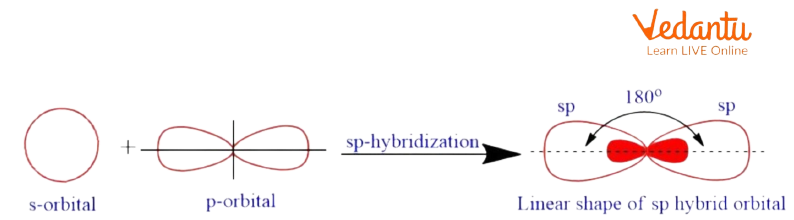Sp Hybridization

• sp² hybridization: it's determined once one s orbital and 2-p orbitals endure a commixture of energy for equivalent orbitals.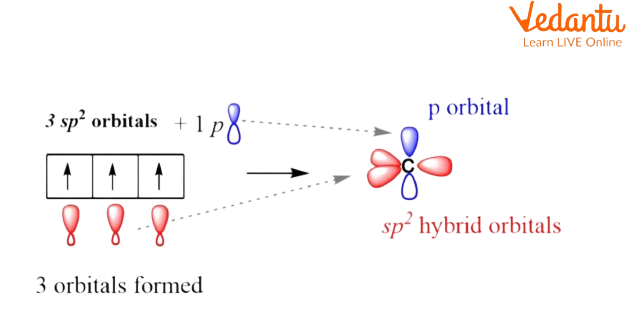sp2 Hybridization.

• sp3 hybridization: once one s orbital and 3-p orbital from the identical atom-shell combine to create a new equivalent orbital, then this is often known as sp³ hybridization.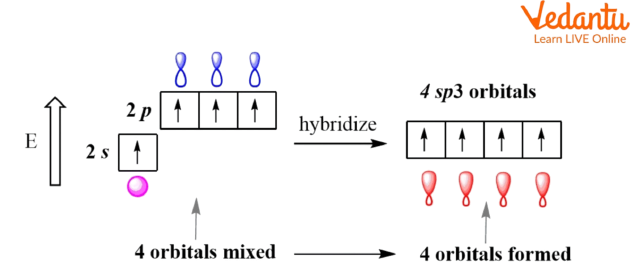sp3 Hybridization.

• sp3d hybridization: It involves the blending of 1-s orbital, 3-p orbitals, and 1-d orbital to create five sp3d hybridized orbitals of equal energy. they need rhombohedral bipyramidal geometry.

• sp3d2 hybridization: It has 1-s, 3-p, and 2-d orbitals, that endure intermixing to create six identical sp3d2 hybrid orbitals.

## Examples

Few examples with different hybridization and shape are discussed below.

1. Berrylium Chloride $\left( \text{BeC}{{\text{l}}_{2}} \right)$

Configuration of Be in ground state: 1s22s2

Since there aren't any odd electrons, it undergoes excitation by promoting one of its 2s electrons into a vacant  2p orbital.

Thus within the excited state, the electronic configuration of Be is 1s2 2s1 2p1.

The discovered form of$\left( \text{BeC}{{\text{l}}_{2}} \right)$  is linear.

Within the excited state, the Be atom undergoes 'sp' hybridization by a combination of 2s and one 2p orbital. Therefore 2 half-filled 'sp' hybrid orbitals are shaped, that are organized linearly.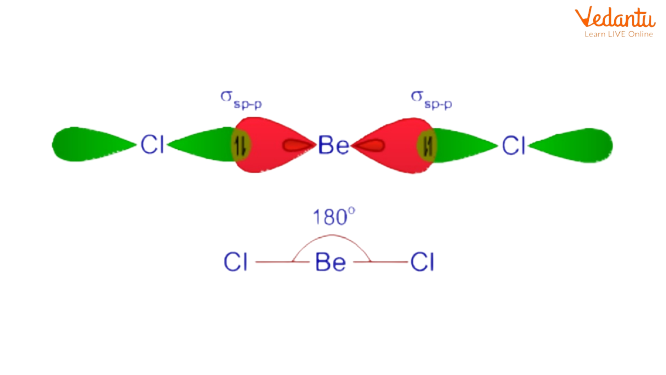Example of Sp Hybridization

2. Methane $\left( \text{C}{{\text{H}}_{\text{4}}} \right)$

During the formation of the alkane molecule, the atom undergoes sp3 hybridizing within the excited state by combining one ‘2s’ and 3 2p orbitals to furnish four half-filled sp3 hybrid orbitals, that are adjusted in tetrahedral symmetry in the area around the atom.

Every sp3 hybrid orbital forms  a ${{\sigma }_{s{{p}^{3}}\pm s}}$ bond with one atom. Therefore carbon forms four${{\sigma }_{s{{p}^{3}}\pm s}}$ bonds with four chemical element atoms.

A methane molecule is tetrahedral in form with ${{109}^{\circ }}28'$ a bond angle.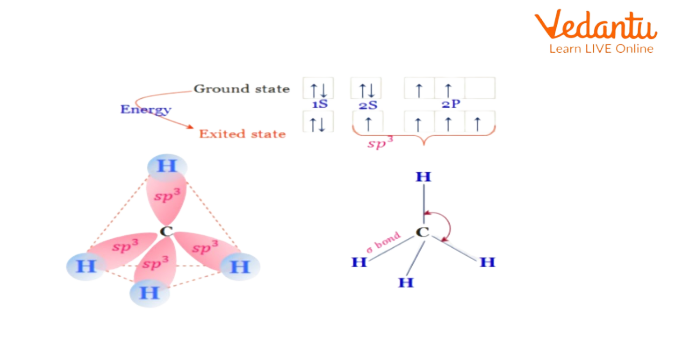Example of sp3 Hybridization

## Conclusion

Hybridization can be explained in chemical science to determine the chemical bonding in cases where the VBT doesn't offer clear assumptions. This theory is specifically used for   determining the bonding in organic molecules. Hybridization is outlined because of the thought of mixing 2 or more atomic orbitals to form a new hybridized orbitals. This intermixing forms hybrid orbitals having completely different energies, shapes, etc.

Last updated date: 27th Sep 2023
Total views: 120.6k
Views today: 1.20k

## FAQs on Hybridization of Atomic Orbitals

1. What is sp hybridization?

The distribution of the energy of the orbitals of individual atoms to allow orbitals of equivalent energy takes place once 2 atomic orbitals mix to make a hybrid orbital in an exceeding molecule is understood as hybridization. The new orbitals formed during this method are referred to as hybrid orbitals. Based on the kinds of orbitals concerned in the mixture, hybridization is often classified into differing kinds like sp hybridization is discovered once 1s and 1p orbital are mixed within the same shell. The new orbital forms are referred to as sp orbitals.

2. What is the hybridization of the central atom in methane?

In methane, carbon has four valence electrons and takes four additional electrons to finish its octet. The four half-filled orbitals of carbon in alkane series before obtaining concern in bond formation, thus their energies are set up and distributed equally to allow four new and equivalent sp3 hybrid orbitals. These four sp3 hybrid orbitals of carbon then overlap with the 1s orbital of four h atoms to allow four s-sp3 bonds. The four bonds in the alkane series are directed toward the four corners of a tetrahedron.

3. What are the exceptions for hybridization in $\text{C}{{\text{O}}_{2}}$ ?

In Carbon dioxide (CO2), the carbon has 2 double bonds, however, it's sp-hybridized. And therefore, the reason for this is often the very fact that the steric range of the carbon is 2 and so as to stay two atoms at 180o, that is that the best pure mathematics, the carbon must use 2 identical orbitals. This is often solely doable within the sp hybridization m. The opposite 2 2p orbitals square measure is used for creating the double bonds on all sides of the carbon. Another common, and really necessary example is carbocations.